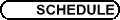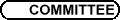SS15 - Topologie de petite dimension et théorie géométrique des groupes / SS15 - Low Dimensional Topology and Geometrical Group TheoryOrg: M. Boileau (Toulouse) et/and S. Boyer (UQAM) S. BASEILHAC, Institut Fourier, Grenoble I, 100 rue des maths, BP 74, 38402 St Martin d'Heres Cedex State sum invariants of flat SL(2,C)-connections on 3-manifolds and the volume conjectures We have recently defined with R. Benedetti a new family of quantum "dilogarithmic" invariants for compact oriented 3-manifolds endowed with a flat SL(2,C)-connection. The quantum dilogarithmic invariants are built via simplicial formula, based on triangulations decorated with a heavy apparatus of combinatorial objects. These can also serve to compute the volume and the Chern-Simons invariant of flat SL(2,C)-connections. In this talk we present some strong structural coincidences in the construction of both family of invariants, and a volume conjecture relating the asymptotic behaviour of the quantum dilogarithmic invariants to the volume and the Chern-Simons invariant of a non compact complete oriented hyperbolic 3-manifold of finite volume. N. BERGERON, Université Paris-Sud, Bat. 425, Orsay 91405 Propriétés de Lefschetz pour les variétés hyperboliques arithmétiques De manière analogue aux théorèmes de Lefschetz pour les variétés projectives, il semble exister des relations entre les groupes de cohomologie d'une variété arithmétique et ceux de ses sous-variétés totalement géodésiques. J'énoncerai, dans le cas des variétés hyperboliques réelles et complexes, une conjecture précise. J'essaierai ensuite de motiver cette conjecture, d'en démontrer certains cas intéressant et d'expliquer ce qu'il advient en rang supérieur. H. BODEN, McMaster University, 1280 Main St. W., Hamilton, ON L8S 4K1, Canada Calculations of the Casson-Curtis SL(2,C) invariant This talk, which presents joint work with Cynthia Curtis, will focus on the SL(2,C) analogue of Casson's invariant. This 3-manifold invariant was defined earlier by Curtis, and we give a simple closed formula for the invariant for Seifert-fibered homology 3-spheres. One way to establish this formula is to use the correspondence between the SL(2,C) character varieties and the moduli spaces of parabolic Higgs bundles of rank two. These results can then be utilized to provide computations for families of 3-manifolds arising as Dehn surgeries on knots with Seifert slopes. For example, we describe computations of the Casson-Curtis for surgeries on twist knots. These computations employ Curtis's surgery formula together with information about the Culler-Shalen seminorms. F. BONAHON, Université de Californie Méridionale Quantum hyperbolic geometry We will discuss a connection between topological quantum field theory and hyperbolic geometry. Following work of Chekhov, Fock and Kashaev, we associate certain non-commutative algebras to manifolds of dimensions 2 and 3. Our main result is that the representation theory of these purely algebraic/combinatorial objects is essentially controlled by the same data as hyperbolic metrics on the corresponding manifolds. We use this construction to exhibit quantum invariants of hyperbolic manifolds. This is joint work with Xiaobo Liu. Nous établissons un lien entre la théorie topologique quantique des champs et la géométrie hyperbolique. A partir de travaux de Chekhov, Fock et Kashaev, nous associons des algèbres non-commutatives aux variétés de dimension 2 et 3. Notre résultat principal est que les représentations de ces objets purement algébriques et combinatoires sont essentiellement controllés par les mêmes données que les métriques hyperboliques sur les variétés correspondantes. Nous utilisons cette construction pour exhiber des invariants quantiques pour les variétés hyperboliques. Ce travail a été effectué en collaboration avec Xiaobo Liu. INNA BUMAGIN, McGill University, Montreal, Quebec, Canada Isomorphism problem for fully residually free, or limit, groups It was proved by O. Kharlampovich and A. Myasnikov that a JSJ decomposition of a limit group can be constructed effectively. To deduce solvability of the isomorphism problem, we show that every automorphism of a limit group preserves an abelian JSJ decomposition of the group (this part is adaptation of joint work with D. Wise). Another ingredient of our proof is a generalization of a theorem proved by Bestvina and by Paulin for hyperbolic groups: using Rips' theory, we show that if G is a rigid vertex group in the JSJ decomposition of a limit group, then G has a finite outer automorphism group modulo edge subgroups. This is joint work with O. Kharlampovich and A. Myasnikov. O. COLLIN, Université du Québec à Montréal Cyclic surgeries, the Alexander polynomial and Floer Homology The goal of this talk is to study which knots in S3 do not admit non-trivial cyclic surgeries or cyclic surgeries with small fundamental group. We will see that the Alexander polynomial contains relevant information for this problem, once it is related to Floer homology of knots. This is work in progress. T. FIEDLER, Université Paul Sabatier, 118 route de Narbonne, Toulouse Simple knots can be detected with polynomial complexity of degree four We introduce a new technique in knot theory which comes from singularity theory. There are two main applications: we give an algorithm which detects the unknot, the trefoil, the figure eight knot, ... with quartic complexity. We construct new calculable knot invariants which are stronger than Vassiliev invariants. BENJAMIN KLAFF, Université du Québec à Montréal Character varieties of hyperbolic 3-manifolds I'll discuss the birational equivalence between the following two useful character varieties associated to a finite-volume hyperbolic 3-manifold M: (1) the "Dehn surgery" component X of the PSL(2,C) character variety of M, and (2) the image of X in the PSL(2,C) variety associated to the boundary components of a compact core for M (under the "restriction" map of characters induced by inclusion of the boundary into M). M. LACKENBY, University of Oxford A characterisation of large finitely presented groups A group is known as "large" if it has a finite index subgroup that admits a surjective homomorphism onto a non-abelian free group. Such groups have many interesting properties, for example super-exponential subgroup growth. Possibly the strongest form of the virtually Haken conjecture asserts that any hyperbolic 3-manifold has large fundamental group. This is known to be true in the cusped case. In my talk, I will give a necessary and sufficient condition for a finitely presented group to be large, in terms of the existence of a nested sequence of finite index subgroups where successive quotients are abelian groups with sufficiently large rank and order. The proof is topological in nature, using a version of thin position for Cayley graphs of finite groups. G. LEVITT, Université de Caen JSJ splittings Jaco-Shalen and Johannson introduced canonical decompositions of 3-manifolds, using tori and annuli. A similar theory has recently been developed for arbitrary finitely presented groups. We describe basic results and applications. S. TILLMANN, UQAM, 201 avenue du Président-Kennedy, Montréal, QC H2X 3Y7, Canada Normal surfaces, angle structures and the character variety I describe geometric splittings of cusped hyperbolic 3-manifolds associated to ideal points of the character variety. The techniques used are geometric and combinatorial, involving normal surfaces, angle structures and hyperbolic geometry. B. WIEST, Université de Rennes I On the complexity of braids We define a measure of "complexity" of a braid which is natural with respect to both an algebraic and a geometric point of view. Algebraically, we modify the standard notion of the length of a braid by introducing generators Dij, which are Garside-like half-twists involving strings i through j, and by counting powered generators Dijk as log(|k|+1) instead of simply |k|. The geometrical complexity is a certain natural measure of the amount of distortion of the n times punctured disk caused by a homeomorphism. Our main result is that the two notions of complexity are comparable. The key rôle in the proofs is played by a technique introduced by Agol, Hass, and Thurston. The methods of our proof, combined with recent work by Hamenstädt, also yield a proof that every braid has a s1-consistent representative of linearly bounded length. Joint with Ivan Dynnikov.